# How to Delete a Node from a Linked List

## How to Delete a Node from a Linked List using C Language:

In this article, I am going to discuss How to Delete a Node from a Linked List using C Programming Language with Examples. Please read our previous article, where we discussed Inserting in a Sorted Linked List using C Language with Examples.

##### Deleting Node From a Linked List:

Here, we will look at the delete operation that is deleting a particular node from a Link List. There are two cases for deleting:

• Delete the first node.
• Delete a node at a given position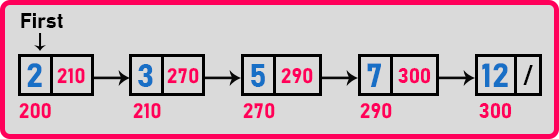In this linked list, ‘first’ is a pointer pointing to the first node. So, if we are deleting the first node that is a special case because if this node is deleted then the next node should become the first node. This is the extra thing that we have to take care of.

In deleting any other node, suppose we want to delete the fourth node then simply delete the node, the first node will not be disturbed, the first remains from the same node. Let us look at case ‘1’: deleting the first node.

##### Case1: Delete the first node in a linked list:

For deleting the first node, we should move the ‘first’ pointer to the next node. If we simply move the pointer to the next node then that becomes the first node and then our linked list starts from the node where ‘first’ is pointing. So, we can access all the other nodes one by one. For a better understanding, please have a look at the below image.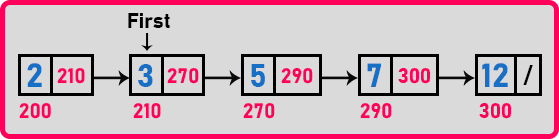Then what about the first node? There is no ‘first’ pointer. So, the first node is not reachable. We cannot go to the first node at all. Nobody is having the address (200) of the first node and hence this node became useless. But it is still there in the main memory. So, this is a very important thing whenever we are deleting a node, make sure to clear the memory allocated for that node. So, when a node is not in use, it must be deleted.

Now let us see how to delete the first node. For accessing the first node, let us point the ‘first’ pointer on the first node as shown in the below image.Now we have to delete this node. For deleting this node, ‘first’ should move to the next node. Then who should delete this node physically from the heap? For deleting this node, we will create one pointer ‘p’ pointing on the first node. For a better understanding, please have a look at the below image.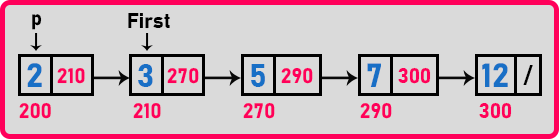Now our linked list starts from the second node where ‘first’ is pointing. ‘3’ node is the starting point of the linked list.

The node where ‘p’ is pointing is no more part of the linked list. So, delete this node from the main memory. By using the ‘first’ pointer we cannot delete it because if we delete it, we will lose the address of the next node. So, we need an extra pointer for the deletion of the node. Let us write down the program code and see how the deletion is done.

##### Delete first node in a linked list pseudo code:

Node *p = first;
first = first->next;
x = p->data;
delete p;

Time Complexity: O(1)

##### Case 2: Delete a node at a given position from a Linked List:

Now let us look at the deletion of any other node at a given position from a linked list. The numbers below in the linked list represent the position of nodes. Let’s say we want to delete the node at the 4th position which is ‘7’.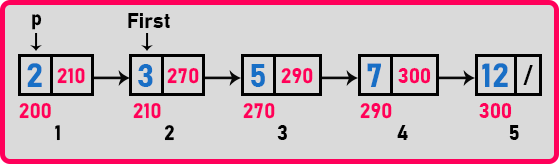Deleting the 4th node from the linked list means that node ‘5’ should point to the ‘12’ node. If the ‘5’ node is pointing on the ‘12’ then automatically ‘7’ node is gone from the linked list.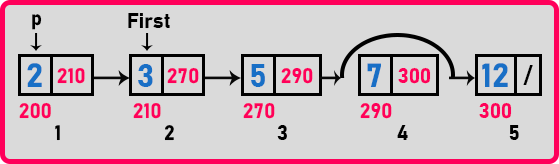Now the node ‘5’ is not pointing to ‘7’. It is pointing to ’12. So now node ‘7’ is not reachable. In this way, the node is deleted. Then also we need to make sure that this node should be physically deleted from the memory. So, we should delete it or deallocate that memory.

For deletion what do we have to do? We have to modify the link of the previous node; in this case, we have to modify the link of the ‘5’ node. And the second thing is we must delete that node physically. How many pointers are required? Two pointers are required. We need a pointer on the previous node for modifying its link and we need a pointer on the node for deallocating from the memory. So, we need two pointers.

Let us see how we can have two pointers. One pointer that we really want on the node which we want to delete and another pointer that we want on the previous node. So, it means one pointer is reaching the node and the other pointer will be following that pointer. We will take ‘q’ as a tail pointer and ‘p’ for the current node pointer as,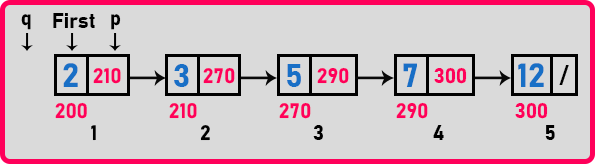Now we will check each and every node and when we found the given node then we will perform the deletion operation. Currently, ‘p’ is on the ‘2’ node but we are searching for the ‘5’ node. So, move ‘q’ and ‘p’ as shown in the below image.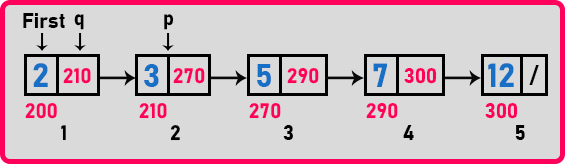Here ‘p’ is pointing to node ‘3’ and ‘q’ is pointing to the previous node i.e. ‘2’. Is ‘p’ is pointing on ‘5’? No, so increment both the pointers as shown in the below image.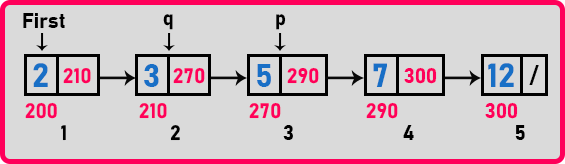Is ‘p’ pointing on node ‘5’? Yes, so ‘p’ is on the node to be deleted and ‘q’ is pointing to the previous node. So how many times we have moved ‘p’? 2 times because we are deleting the node in the 3rd position. So, for any position ‘k’ we should move it for ‘k-1’ time. We will delete the link of the ‘q’ node and point it to ‘p->next’ as,Now let us write the pseudo-code for this.

##### Delete a node at a given position from a Linked List Pseudo Code:

Node *p = first;
Node *q = NULL;
for(i = 0; i < pos-1; i++){
q = p;
p = p->next;
}
q->next = p->next;
x = p->data;
free(p);

##### Analysis:

How many links have been modified? Just one link has been modified. No change in the node because that node will be deleted. So, there’s no modification of the link. How many pointers are required? Two pointers ‘p’ and ‘q’ are for deleting the node.

What is the time taken? The time span here is only in the ‘for’ loop. So, the time taken by the loop depends on the position that we are giving. If we are giving the second position then the time is constant, and ‘p’ and ‘q’ do not move at all. If we are giving the last position that the time is maximum.

Time taken:
Minimum: O (1)
Maximum: O (n)

Now we will write a single function that will take the index and delete that node whether it may be a first node or any of that node

##### Function for deleting a node in a linked list:
```int Delete(int pos){
Node *p, *q;
int x = -1, i;

if(pos == 1){
x = first->data;
p = first;
first = first->next;
free(p);
}
else{
p = first;
q = NULL;
for(i = 0; i < pos - 1 && p; i++){
q = p;
p = p->next;
}
if(p){
q->next = p->next;
x = p->data;
free(p);
}
}
return x;
}
```

Now let us write a complete program to delete a particular node in a given linked list using C Programming Language.

##### Program for Deleting from Linked List in C Language
```#include <stdio.h>
#include <stdlib.h>

struct Node
{
int data;
struct Node *next;
}   *first = NULL;

void Create(int A[], int n)
{
int i;
struct Node *t, *last;
first = (struct Node *) malloc(sizeof(struct Node));
first->data = A;
first->next = NULL;
last = first;

for (i = 1; i < n; i++)
{
t = (struct Node *) malloc(sizeof(struct Node));
t->data = A[i];
t->next = NULL;
last->next = t;
last = t;
}
}

int Count(struct Node *p)
{
int l = 0;
while(p)
{
l++;
p = p->next;
}
return l;
}

void Display(struct Node *p)
{
while(p != NULL)
{
printf ("%d ", p->data);
p = p->next;
}
}

int Delete(int pos)
{
struct Node *p, *q;
int x = -1, i;

if (pos == 1)
{
x = first->data;
p = first;
first = first->next;
free(q);
}
else
{
p = first;
q = NULL;
for (i = 0; i < pos - 1 && p; i++)
{
q = p;
p = p->next;
}
if (p)
{
q->next = p->next;
x = p->data;
free(p);
}
}
return x;
}

void Insert(struct Node *p, int index, int x)
{
struct Node *t;
int i;

if (index < 0 || index > Count(p))
return;
t = (struct Node *) malloc (sizeof (struct Node));
t->data = x;

if (index == 0)
{
t->next = first;
first = t;
}
else
{
for (i = 0; i < index - 1; i++)
p = p->next;
t->next = p->next;
p->next = t;

}
}

int main()
{
int A[] = { 8, 3, 7, 12 };
Create(A, 4);
printf ("Before Delete: ");
Display (first);
Delete (2);
printf ("\nAfter Delete: ");
Display (first);
return 0;
}
```
###### Output: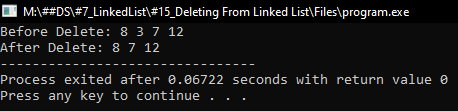In the next article, I am going to discuss How to Check if a Linked List is Sorted in C Language with Examples. Here, in this article, I try to explain How to Delete a Node from a Linked List in C Language with Examples and I hope you enjoy this Deleting from Linked List in C Language with Examples article.